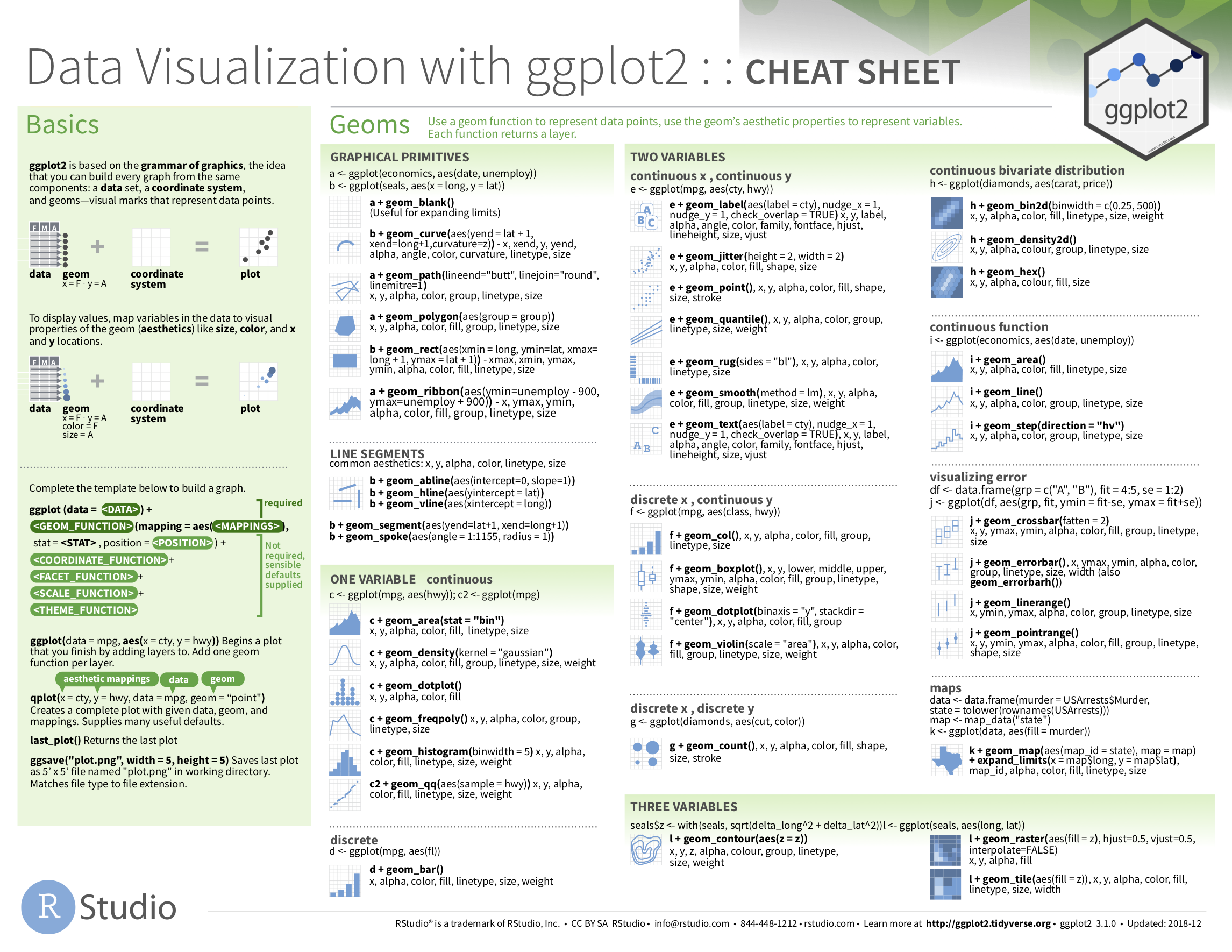## 2.9 Conclusion

### 2.9.1 Summary table

Let’s recap all five of the five named graphs (5NG) in Table 2.4 summarizing their differences. Using these 5NG, you’ll be able to visualize the distributions and relationships of variables contained in a wide array of datasets. This will be even more the case as we start to map more variables to more of each geometric object’s aesthetic attribute options, further unlocking the awesome power of the ggplot2 package.

TABLE 2.4: Summary of Five Named Graphs
Named graph Shows Geometric object Notes
1 Scatterplot Relationship between 2 numerical variables geom_point()
2 Linegraph Relationship between 2 numerical variables geom_line() Used when there is a sequential order to x-variable, e.g., time
3 Histogram Distribution of 1 numerical variable geom_histogram() Facetted histograms show the distribution of 1 numerical variable split by the values of another variable
4 Boxplot Distribution of 1 numerical variable split by the values of another variable geom_boxplot()
5 Barplot Distribution of 1 categorical variable geom_bar() when counts are not pre-counted, geom_col() when counts are pre-counted Stacked, side-by-side, and faceted barplots show the joint distribution of 2 categorical variables

### 2.9.2 Function argument specification

Let’s go over some important points about specifying the arguments (i.e., inputs) to functions. Run the following two segments of code:

# Segment 1:
ggplot(data = flights, mapping = aes(x = carrier)) +
geom_bar()

# Segment 2:
ggplot(flights, aes(x = carrier)) +
geom_bar()

You’ll notice that both code segments create the same barplot, even though in the second segment we omitted the data = and mapping = code argument names. This is because the ggplot() function by default assumes that the data argument comes first and the mapping argument comes second. As long as you specify the data frame in question first and the aes() mapping second, you can omit the explicit statement of the argument names data = and mapping =.

Going forward for the rest of this book, all ggplot() code will be like the second segment: with the data = and mapping = explicit naming of the argument omitted with the default ordering of arguments respected. We’ll do this for brevity’s sake; it’s common to see this style when reviewing other R users’ code.

An R script file of all R code used in this chapter is available here.

If you want to further unlock the power of the ggplot2 package for data visualization, we suggest that you check out RStudio’s “Data Visualization with ggplot2” cheatsheet. This cheatsheet summarizes much more than what we’ve discussed in this chapter. In particular, it presents many more than the 5 geometric objects we covered in this chapter while providing quick and easy to read visual descriptions. For all the geometric objects, it also lists all the possible aesthetic attributes one can tweak. In the current version of RStudio in late 2019, you can access this cheatsheet by going to the RStudio Menu Bar -> Help -> Cheatsheets -> “Data Visualization with ggplot2.” You can see a preview in the figure below.FIGURE 2.28: Data Visualization with ggplot2 cheatsheet.

### 2.9.4 What’s to come

Recall in Figure 2.2 in Section 2.3 we visualized the relationship between departure delay and arrival delay for Alaska Airlines flights. This necessitated paring down the flights data frame to a new data frame alaska_flights consisting of only carrier == AS flights first:

alaska_flights <- flights %>%
filter(carrier == "AS")

ggplot(data = alaska_flights, mapping = aes(x = dep_delay, y = arr_delay)) +
geom_point()

Furthermore recall in Figure 2.7 in Section 2.4 we visualized hourly temperature recordings at Newark airport only for the first 15 days of January 2013. This necessitated paring down the weather data frame to a new data frame early_january_weather consisting of hourly temperature recordings only for origin == "EWR", month == 1, and day less than or equal to 15 first:

early_january_weather <- weather %>%
filter(origin == "EWR" & month == 1 & day <= 15)

ggplot(data = early_january_weather, mapping = aes(x = time_hour, y = temp)) +
geom_line()

These two code segments were a preview of Chapter 3 on data wrangling using the dplyr package. Data wrangling is the process of transforming and modifying existing data with the intent of making it more appropriate for analysis purposes. For example, these two code segments used the filter() function to create new data frames (alaska_flights and early_january_weather) by choosing only a subset of rows of existing data frames (flights and weather). In the next chapter, we’ll formally introduce the filter() and other data wrangling functions as well as the pipe operator %>% which allows you to combine multiple data wrangling actions into a single sequential chain of actions. On to Chapter 3 on data wrangling!